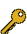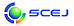## S-25. \$BG.9)3X%7%s%]%8%&%`(B

 \$B%*!<%,%J%\$%6!<(B \$BCfA>9@0l!J6e=#Bg!K!&c7F#BYMN!JElKLBg!K!&F|=P4V\$k\$j!J?@8MBg!K(B

\$BK\%7%s%]%8%&%`\$G\$OG.\$rpJs8r49\$r9T\$\$\$J\$,\$i!\$;}B32DG=\$J

\$B:G=*99?7F|;~!'(B2014-08-04 11:49:01\$B\$3\$NJ,N`\$G\$h\$/;H\$o\$l(B
\$B\$F\$\$\$k%-!<%o!<%I(B
\$B%-!<%o!<%I(B\$B
Heat Transfer4\$B7o(BFluidized Bed2\$B7o(BPM2\$B7o(Bsurfactant1\$B7o(B\$B\$BHV9f(B\$B9V1iBjL\!?H/I=\$B%-!<%o!<%I(B\$B
9[\$BM%=(O@J8>^(B]\$B@PC:%A%c!
(\$B4tIlBg9)(B) \$B!{(B(\$B@5(B)\$BHDC+(B \$B5A5*(B\$B!&(B (\$BL>Bg1!9)(B) (\$B@5(B)\$B9b:d(B \$BJ8:H(B\$B!&(B (\$B4tIlBg9)(B) (\$B@5(B)\$B>.NS(B \$B?.2p(B
Coal Char Particles
Extinction efficiency
4/22
13:26:01
58\$B%^%\$%/%mGH>H
(\$BJ<8K8)Bg9)(B) (\$B3X(B)\$BCfED(B \$BNC2p(B\$B!&(B (\$B3X(B)\$B6;1J(B \$BBsLi(B\$B!&(B (\$B3X(B)\$B>>B<(B \$B=S8c(B\$B!&(B \$B!{(B(\$B@5(B)\$BD+7'(B \$BM52p(B
microwave
DLS
nano-bubble
5/1
09:49:55
74[\$BE8K>9V1i(B]\$BG3>F\$H:`NA9g@.(B
(\$BKLBg(B) \$B!{(B(\$B@5(B)\$B=);3(B \$BM'9((B
Combustion Synthesis
Energy Saving
SiAlON
5/1
16:05:24
76[\$BE8K>9V1i(B]\$B8:05\$5\$l\$?JD6u4V\$K\$*\$1\$k<+A3BPN.(B
(\$B2,;3M}Bg9)(B) \$B!{(B(\$B@5(B)\$BJ?Ln(B \$BGnG7(B
natural convection
heat transfer
low pressure
5/1
16:11:50
241\$BHy>.=ENO4D6-2<\$K\$*\$1\$k(BTLZ\$BK!\$K\$h\$k6Q0lAH@.(BSiGe\$B7k>=@.D9>r7o\$N?tCM2r@OE*8!F\$(B
(\$BElKLBg1!9)(B) \$B!{(B(\$B3X(B)\$B=;2,(B \$B:;Me(B\$B!&(B (\$B3X(B)\$B0\$It(B \$B7IB@(B\$B!&(B (\$B@5(B)\$B?y2,(B \$B7r0l(B\$B!&(B (\$B@5(B)\$B5WJ](B \$B@5\$B!&(B (\$B@5(B)\$BDMED(B \$BN4IW(B\$B!&(B (JAXA) \$BLZ2<(B \$B630l(B\$B!&(B \$B9S0f(B \$B9/CR(B\$B!&(B \$B0pIY(B \$BM58w(B
SiGe crystal growth
TLZ method
Numerical simulation
5/9
12:35:21
253\$BGvKlFb(B Marangoni \$BBPN.\$NN.F0J}8~\$KBP\$9\$k1UKl7A>u\$H12\$N4X78@-\$N1F6A\$K4X\$9\$k?tCM2r@O(B
(\$B:eBg1!4p9)(B) \$B!{(B(\$B3X(B)\$B;3K\(B \$BBnLi(B\$B!&(B (\$B@5(B)\$B9bLZ(B \$BMNJ?(B\$B!&(B (\$B@5(B)\$B2,Ln(B \$BBYB'(B
Thin film
Dynamics of vortex
Marangoni effect
5/9
13:43:14
504\$BH?1~O'Fb%5%s%W%j%s%0\$K\$h\$k%+!<%\%s%J%NN3;R\$N@.D95sF0\$N8!F\$(B
(\$BElKLBg1!9)(B) \$B!{(B(\$B3X(B)\$BEOJU(B \$B0&5.(B\$B!&(B (\$B3X(B)\$B>.Ln(B \$B8xFA(B\$B!&(B \$B=P1)(B \$B0l\$B!&(B (\$B3X(B)\$B>>@n(B \$B2ELi(B\$B!&(B (\$B@5(B)\$Bc7F#(B \$BBYMN(B\$B!&(B (\$B@5(B)\$B>>2<(B \$BMN2p(B\$B!&(B (\$B@5(B)\$B@DLZ(B \$B=(G7(B\$B!&(B (\$B00%+!<%\%s(B) (\$BK!(B)\$B9>NI(B \$B9/5.(B\$B!&(B (\$BK!(B)\$B@DLZ(B \$B?r9T(B\$B!&(B (\$B@5(B)\$B;38}(B \$BEl8c(B
carbonblack
soot
SMPS
5/12
10:06:25
572\$BDL5\$F0NODc8:\$K\$h\$k5[Ce<0>x5\$@8@.%R!<%H%]%s%W\$N9b8zN(2=(B
(\$B6eBg1!9)(B) \$B!{(B(\$B3X(B)\$B>.NS(B \$B=S2p(B\$B!&(B \$BEDCf(B \$BM\$\$B!&(B (\$B3X(B)\$B9>Eg(B \$B>"B@O:(B\$B!&(B (\$B6eBg9)(B) (\$B@5(B)\$BCfA>(B \$B9@0l(B\$B!&(B (\$B@5(B)\$B?<0f(B \$B=a(B
heat pump
packed bed
pressure drop
5/12
12:16:02
583\$B@E<'>l=E>vEE<'IbM7K!\$rMxMQ\$7\$?MOM;(BCuCo\$B9g6b\$N?bD>J,8wJ|
(\$BElKLBg1!9)(B) \$B!{(B(\$B3X(B)\$B>eLn(B \$BfFLi(B\$B!&(B (\$B3X(B)\$B9u_7(B \$BN<(B\$B!&(B (\$B3X(B)\$BKL86(B \$BMc(B\$B!&(B (\$B@5(B)\$B?y2,(B \$B7r0l(B\$B!&(B (\$B@5(B)\$B5WJ](B \$B@5\$B!&(B (\$B@5(B)\$BDMED(B \$BN4IW(B\$B!&(B (\$BElKLBgB?858&(B) \$BBG1[(B \$B2m?N(B\$B!&(B \$BJ!;3(B \$BGnG7(B
molten CuCo
Electromagnetic levitation
Normal spectral emissivity
5/12
12:41:09
632\$B%<%*%i%\$%H\$rMQ\$\$\$?5[Ce<0>x5\$@8@.%7%9%F%`\$K\$*\$1\$k>x5\$@8@.4o7A>u\$N8!F\$(B
(\$B2,;38)Bg1!(B) \$B!{(B(\$B3X(B)\$B@>(B \$B5.Bg(B\$B!&(B (\$B@5(B)\$BG=EPO)(B \$BM5(B\$B!&(B (\$B2,;38)Bg(B) (\$B@5(B)\$BCf@n(B \$BFsI'(B
Zeolite
Steam generator
5/12
14:27:10
671\$BI9E@2
(\$BH,9)Bg1!(B) \$B!{(B(\$B3X(B)\$B;3ED(B \$BD>\$B!&(B (\$BH,9)Bg(B) (\$B@5(B)\$BLnED(B \$B1QI'(B\$B!&(B (\$B@5(B)\$B9b66(B \$B?8(B\$B!&(B \$B>.EDEg(B \$BAo(B
Kinematic viscosity
absorption refrigerator
working liquid
5/12
15:52:10
706\$B7\2A(B
(\$B4tIlBg(B) \$B!{(B(\$B3X(B)\$B0KF#(B \$BBg51(B\$B!&(B (\$B@5(B)\$B>.NS(B \$B?.2p(B\$B!&(B (\$B@5(B)\$BHDC+(B \$B5A5*(B\$B!&(B (\$B%O%\$%F%`(B) \$BDX0f(B \$B9/;J(B
heat transfer
heat insulating material
5/12
16:51:36
715\$B3&LL3h@-:^\$rE:2C\$7\$?@xG.M"Aw%9%i%j!<\$NN.F0!&EAG.\$K4X\$9\$k%5%\$%:8z2L%b%G%k(B
(\$B?@8MBg1!9)(B) \$B!{(B(\$B@5(B)\$BNkLZ(B \$BMN(B\$B!&(B (\$B?@8MBg1!(B) (\$B3X(B)\$BEDCf(B \$B9'Fs(B\$B!&(B (\$B?@8MBg<+(B) (\$B@5(B)\$BF|=P4V(B \$B\$k\$j(B\$B!&(B (\$B?@8MBg1!9)(B) (\$B@5(B)\$B8VED(B \$B1YG7(B
Latent Heat Transportation
Friction Coefficient
Heat Transfer
5/12
17:02:35
749\$B%3%s%Q%/%H7?%W%l!<%H%U%#%s<0G.8r494o\$K\$*\$1\$kEAG.FC@-2r@O(B
(\$B;:Am8&(B) \$B!{(B(\$B@5(B)\$B1J2'(B \$BN60l(B
Heat Exchanger
Heat Transfer
Computational Fluid Dynamics
5/12
17:32:29
825\$B3&LL3h@-:^\$rE:2C\$7\$?=-2=%j%A%&%`?eMO1U%^%i%s%4%KBPN.\$K4X\$9\$k8&5f(B
(\$B?@8MBg1!(B) \$B!{(B(\$B3X(B)\$B_7(B \$B>04u(B\$B!&(B (\$B?@8MBg<+(B) (\$B@5(B)\$BF|=P4V(B \$B\$k\$j(B\$B!&(B (\$B?@8MBg1!9)(B) (\$B@5(B)\$BNkLZ(B \$BMN(B\$B!&(B (\$B@5(B)\$B8VED(B \$B1YG7(B
Marangoni
absorption
surfactant
5/12
18:44:07
892\$BN.F0AX<0(BPM\$B=|5nAuCV\$N(BPM\$BJa=8FC@-\$K4X\$9\$k?tCM2r@O(B
(\$B6eBg1!9)(B) \$B!{(B(\$B3X(B)\$BDZ0f(B \$B5.9-(B\$B!&(B (\$B@5(B)\$B;3K\(B \$B9d(B\$B!&(B (\$B@5(B)\$B?<0f(B \$B=a(B\$B!&(B (\$B@hC<5;8&(B) (\$B@5(B)\$B4\NS(B \$BWv(B
PM
Fluidized Bed
5/12
19:54:00
962\$BN.F0AX\$rMQ\$\$\$?O"B3:F@8<0(BPM\$B=|5nAuCV\$K\$*\$1\$k%+%j%&%`E:2C\$N1F6A(B
(\$B6eBg1!9)(B) \$B!{(B(\$B3X(B)\$BFo(B \$B979b(B\$B!&(B (\$B@5(B)\$B;3K\(B \$B9d(B\$B!&(B (\$B@5(B)\$B?<0f(B \$B=a(B\$B!&(B (\$B@hC<5;8&(B) (\$B@5(B)\$B4\NS(B \$BWv(B
PM
Fluidized Bed
Kalium
5/12
21:33:12
994\$BL\$MxMQ29GSG.\$rMxMQ\$7\$?5[<}<0%R!<%H%]%s%W\$N@8;:%W%m%;%9\$X\$N3hMQ5;=Q(B
(\$B?9>>9)6H(B) \$B!{(B(\$B@5(B)\$B4]LS(B \$B8,\$B!&(B (\$B4tIlBg9)(B) (\$B@5(B)\$B>.NS(B \$B?.2p(B\$B!&(B (\$B@5(B)\$BHDC+(B \$B5A5*(B
Absorption heat pump system
Waste hot water
Productive process
5/12
22:15:19

\$B9V1i?=\$79~\$_0lMw(B(\$B%7%s%]%8%&%`HV9f!&9V1iJ,N`HV9fJL(B)

\$B
\$B2=3X9)3X2q(B \$BBh(B46\$B2s=)5(Bg2q(B(C) 2014 \$B8x1W
Most recent update: 2014-08-04 11:49:01
E-mail: inquiry-46fwww3.scej.org
This page was generated byeasp 2.34; update.pl 2.33 (C)1999-2013 kawase